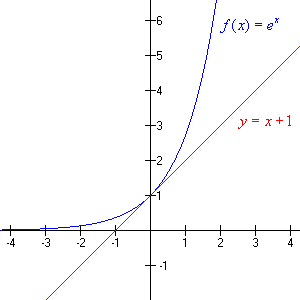# The Number $e$

The number, $e$ , is an irrational number defined as the limit of $x$ as $x$ approaches infinity of ${\left(1+\frac{1}{x}\right)}^{x}$ , with a decimal expansion beginning $2.7182818284590452353602874713527...$ . It is an important number in calculus, defined so that the slope of the line tangent to $f\left(x\right)={e}^{x}$ at $\left(0,1\right)$ is equal to $1$ .

Because $f\left(x\right)={e}^{x}$ has special calculus properties that simplify many calculations, it is considered the natural exponential function .